Email us to get an instant 20% discount on highly effective K-12 Math & English kwizNET Programs!

#### Online Quiz (WorksheetABCD)

Questions Per Quiz = 2 4 6 8 10

### Geometry7.3 Reflections

 Reflection of (x, y) in x-axis is (x, -y)Reflection of (x, y) in the y-axis is (-x, y)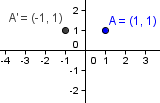Reflection uses a line of reflection to create a mirror image of the original figure.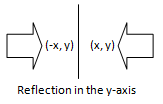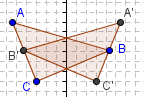Coordinate rules for Reflections: If (a,b) is reflected in the x-axis, its image is the point (a, -b) If (a,b) is reflected in the y-axis, its image is the point (-a, b) If (a,b) is reflected in the line y = x, its image is the point (b, a) If (a,b) is reflected in the line y = -x, its image is the point (-b, -a) Directions: Solve the following problems. Use a graph paper to graph the reflection of the following polygon: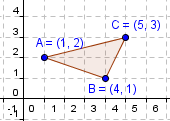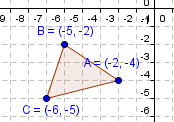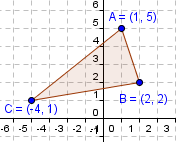Also write at least 5 examples of your own.
 Q 1: The image of (-1, 2) in the line y=-x is ______.(1,2)(-1,2)(-2,1) Q 2: The image of (1,2) in the Y-axis is ______.(-1,-2)(-1,2)(1,-2) Q 3: The image of (-4,-3) in the X-axis is ______.(-4,3)(4,-3)(4,3) Q 4: The image of (-4,6) in the Y-axis is ______.(4,-6)(4,6)(-4,-6) Q 5: The image of (5,-4) in the Y-axis is ______.(5,4)(-5,-4)(-5,4) Q 6: The image of (1,2) in the X-axis is ______.(-1,2)(-1,-2)(1,-2) Question 7: This question is available to subscribers only! Question 8: This question is available to subscribers only!